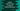# How to insert an item to the start of an Ordered dictionary in Python## How to insert an item to the start of an Ordered dictionary in Python:

Using python dictionary, we can store key-value pairs. Using the key, we can get the value stored for that key. There is another subclass of dictionary called OrderedDict. The difference between dict and OrderedDict is that OrderedDict remembers the order the key-value pairs are inserted, but a normal dictionary doesn’t.

In this post, we will learn how to insert an item to an Ordered dictionary in the beginning, or we will add one item as the first element.

## Two ways to solve this:

We can solve this in two different ways. The first one is simple. Create one new dictionary with the new key-value pairs and the old dictionary key-value pairs. We will add the new key-value pairs at the start.

Another way is to use the update method of OrderedDict class that can be used to update any pairs in an ordered dictionary.

### Method 1: By creating a new OrderedDict:

Let’s try to solve this by creating a new OrderedDict. Below is the complete program:

``````from collections import OrderedDict

givenDict = OrderedDict([('zero', 0), ('one', 1)])
print('Original Dictionary : {}'.format(givenDict))

newPairs = OrderedDict([('count', 2)])

newDict = OrderedDict(list(newPairs.items()) + list(givenDict.items()))

print('New Dictionary : {}'.format(newDict))``````

Here,

• givenDict is the given ordered dictionary with two key-value pairs. We are printing the content of this dictionary at the start of the program.
• newPairs is the key-value pairs to add to the start of the givenDict.
• newDict is the newly created dictionary by adding the newPairs to the start and givenDict to the end.
• The last line is printing the contents of the newDict.

If you run this program, it will print the below output:

### Method 2: By using update and move_to_end:

We can also use update and move_to_end methods. These methods are defined in the OrderedDict class. By using update, we can add one key-value pairs to the end of the dictionary and using move_to_end, we can move that pair to the start of the dictionary.

Below is the complete program:

``````from collections import OrderedDict

givenDict = OrderedDict([('zero', 0), ('one', 1)])
print('Original Dictionary : {}'.format(givenDict))

givenDict.update({'count': 2})
givenDict.move_to_end('count', last=False)

print('New Dictionary : {}'.format(givenDict))``````

This program will print the same output.

``````Original Dictionary : OrderedDict([('zero', 0), ('one', 1)])
New Dictionary : OrderedDict([('count', 2), ('zero', 0), ('one', 1)])``````

The advantage of this method is that we don’t have to create one intermediate dictionary object.